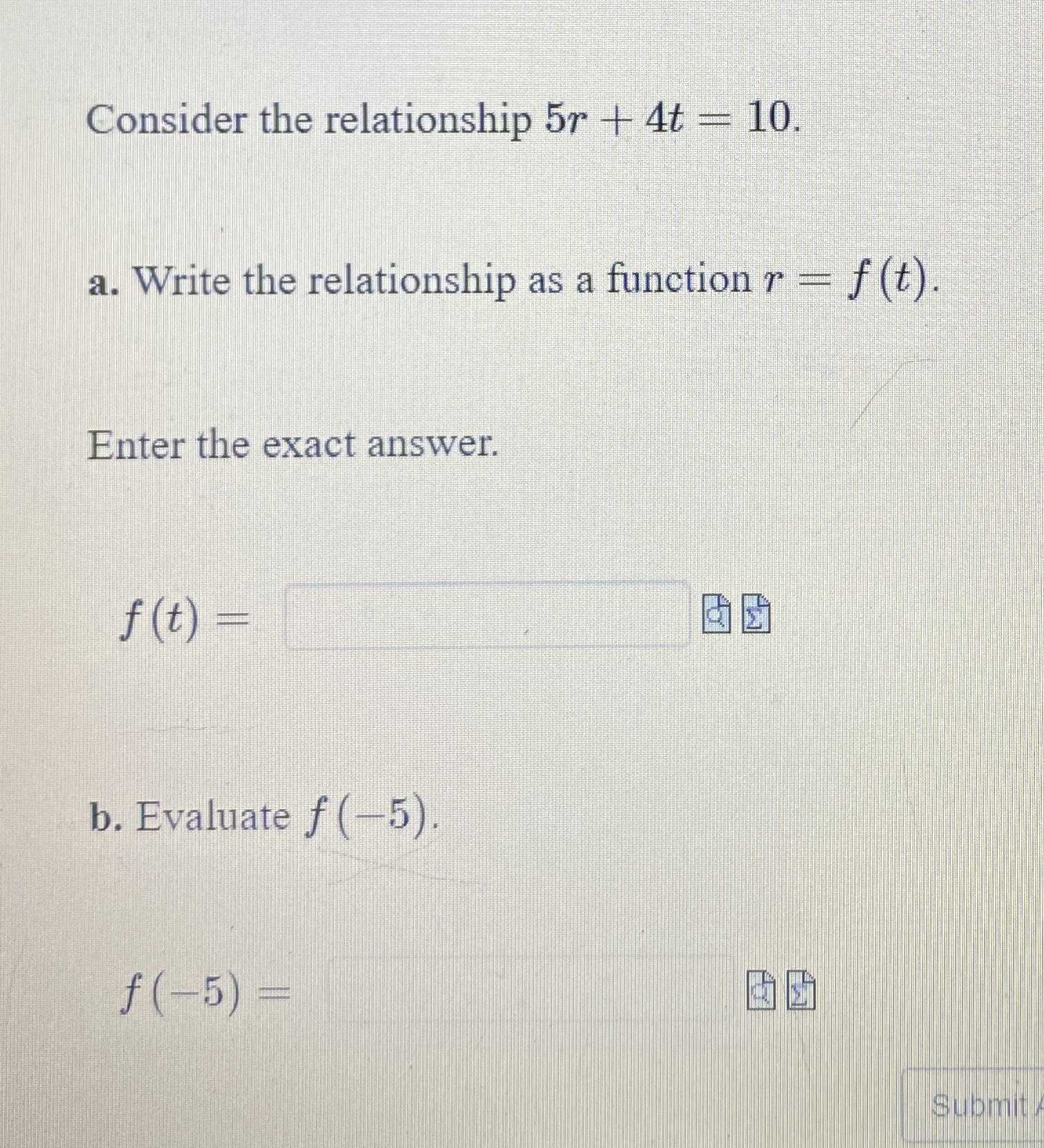### ¿Todavía tienes preguntas de matemáticas?

Pregunte a nuestros tutores expertos
Algebra
PreguntaConsider the relationship $$5 r + 4 t = 10 .$$

a. Write the relationship as a function $$r = f ( t )$$ . Enter the exact answer.

$$f ( t ) =$$

b. Evaluate $$f ( - 5 )$$ .

$$f ( - 5 ) =$$

$$f(t)= 2- 0.8t\\f(- 5)= 6$$